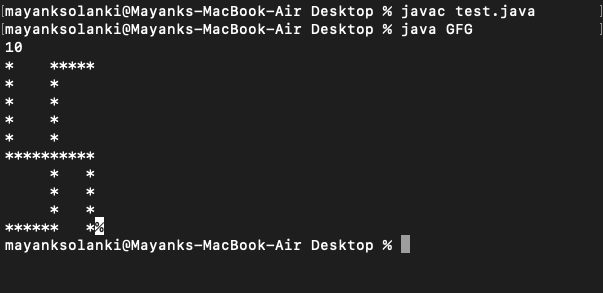Java Program to Print Swastika Sign By Taking User Input

• Difficulty Level : Easy
• Last Updated : 31 Aug, 2021

Take as input N, an odd number (>=5). Print the following pattern as given below for N = 7. Here the input format is the value of N and output results out in swastika pattern printing.

Illustration:

Input Format: Enter value of N ( >=5 )
Constraints: 5 <= N <= 99
Output Format: Print the required pattern.
*     * * * *

*     *

*     *

* * * * * * *

*     *

*     *

* * * *       *

Let us thinks of a way out in order to figure out by listing the logic prior to writing any code in order to generate the desired pattern.

Procedure:

1. First, take an input (N) from the user.
2. Initialize rows and cols as 1 to make loops in for the statement.
3. In the first row, we definitely know we have to print a * then give some spaces till N/2, and then again print stars till N.
4. In the second to N/2 rows, first, we need to print a * then spaces till N/2 (that it , we don’t need to provide spaces here)
5. In the (N/2)+1 th row, just print * till N.
6. Again in (N/2) +2th row to N -1, we need to first print spaces till N/2 and then print a * and the spaces till N-1 and finally a * at the end
7. In the final row, we need to print * till (N/2) + 1 and then print spaces till N-1 now and then finally ending it a *.
8. Lastly do not forget to add System.out.println() to add a blank line after each line of work

Now let us implement the some by writing the core for the same.

Example

Java

 // Java program to Illustrate Swastika Sign Pattern Printing  // Main class// SwastikaSignclass GFG {      // Main driver method    public static void main(String args[])    {          // Creating an object of Scanner clas to read input        // from user        Scanner sc = new Scanner(System.in);          // Reading number N from user        int N = sc.nextInt();        // Custom setting the rows to be 10 to        // understand the output          // Initializing rows to unity initially        int rows = 1;          // This condition holds true        // Till rows are lesser than input number from user        while (rows <= N) {              if (rows == 1) {                  // Start work                System.out.print("*");                  // Space work                for (int csp = 1; csp < N / 2; csp++)                    System.out.print(" ");                  // Star work                for (int cst = (N / 2) + 1; cst <= N; cst++)                      System.out.print("*");                System.out.println();            }              else if (rows <= N / 2 && rows > 1) {                // Star work                System.out.print("*");                  // Space work                for (int csp = 1; csp < N / 2; csp++)                      System.out.print(" ");                System.out.print("*");                System.out.println();            }              else if (rows == (N / 2) + 1) {                  for (int cstt = 1; cstt <= N; cstt++)                      System.out.print("*");                System.out.println();            }              else if (rows <= N - 1 && rows > (N / 2) + 1) {                  // Space work                for (int csp = 1; csp <= N / 2; csp++)                    System.out.print(" ");                  // Star work                System.out.print("*");                  // Space work                for (int cspp = 1 + (N / 2); cspp < N - 1;                     cspp++)                      System.out.print(" ");                  // Star work                System.out.print("*");                System.out.println();            }            else {                  for (int csttt = 1; csttt <= (N / 2) + 1;                     csttt++)                      System.out.print("*");                  for (int csppp = (N / 2) + 2;                     csppp <= N - 1; csppp++)                      System.out.print(" ");                System.out.print("*");            }            rows++;        }    }}

Output:Note: One can also use BufferedReader class if you want to take input from user to enter the number of rows here.

My Personal Notes arrow_drop_up